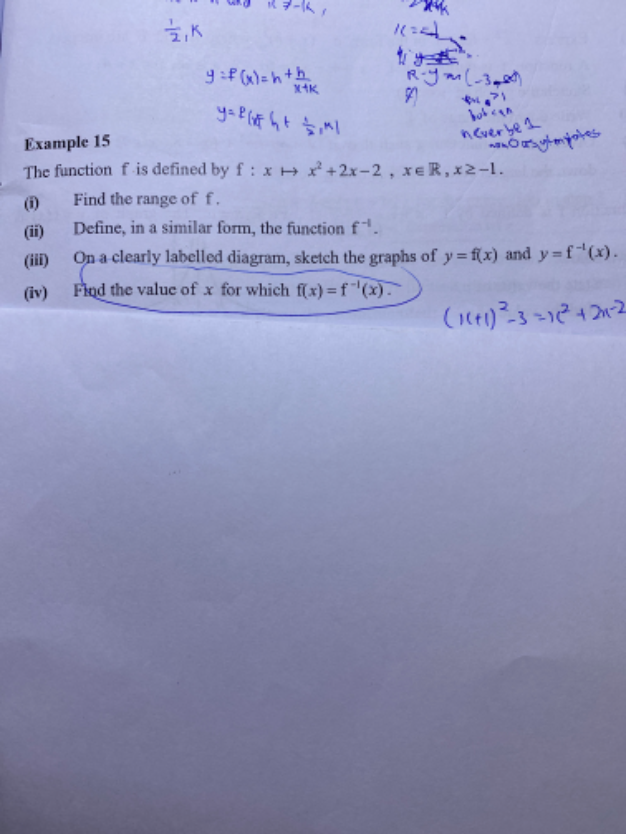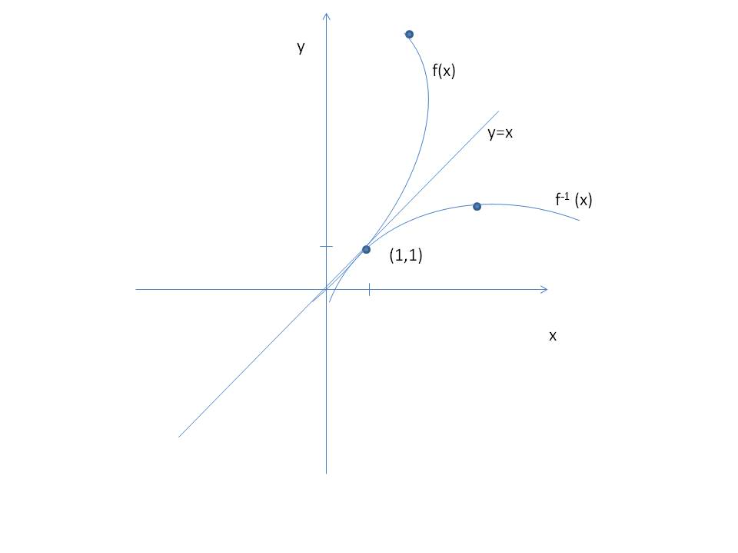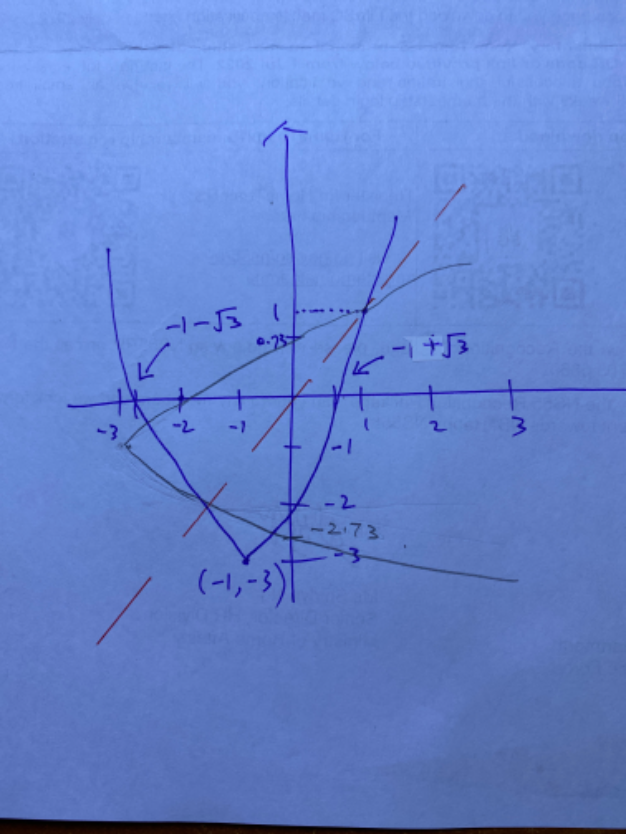# QuestionKindly help on this question thanks

Quoting you:

i) (x+1)^2-3, so the range of y is -3,

sub x >= -1 into x^2-2x-2 = 1, so wouldn’t the range for y is [-3,1]?

Sorry i don’ t have the answer, so correct me if i am wrong.

Equation in blue is wrong.

f(x) = y  =  (x+1)^2-3 = x^2+2x-2

So if you substitute a range of x ≥ -1,

x         -1           0           1          2         3        4     ………………………

y         -3          -2          1          6         13      22    etc….

Hence the range where y lies is [-3, ∞ )

Sorry I typed it the other way round earlier in haste, negative number comes first.

Quoting you:

iii) please look at my sketching, is it correct? is a mirror effect of x^2+2x-2. so when x>=-1 i can see only one point (1,1) meet, therefore you say x value is 1?

Your sketch is correct but the range for x ≥ -1 only.  So it should not include points outside this range in the sketch.   (However, you can draw the whole parabola to see the effect of the 2 parabolas, one vertical and one horizontal, that means your inverse function is correctly derived.)

I only sketched partially because of the range and it was tough to draw it digitally as well.

I see your sketch has another point of intersection at x = -0.xxx, y =-2.xxx ?  I cannot be sure if that point is accurate, perhaps you should use a graph paper to try.  My simple thinking is if they want you to identify a point from a sketch(i.e. not a graph), the answer has to be something easy and obvious to read on the sketch like the clear intersection point (1,1).  And if there are other points of intersection, it would be outside  x ≥ -1 hence the value of x would not be valid.

Thanks very much for your explanation. 🙂

0 Replies 0 Likesf(x) = x2 + 2x – 2   x  ≥ -1

(i)  x= -1

f(-1) = (-1)2 + 2(-1) – 2 = -3

Range for f(x) = (∞, -3]

(ii)   y = x2 + 2x – 2

=  (x2 + 2x  + 1) -1 – 2

=  (x+1)2 -3

x = (y+1)2 -3

(y+1)2  = x + 3

y + 1 =  ± sqrt(x+3)

y = f-1 (x) = -1 ± sqrt(x+3)

Since x ≥-1, in the inverse function, y ≥ -1, so  y = f-1 (x) = -1 + sqrt(x+3)

(iii)  See diagram attached

The graph of f(x) and  f-1 (x) are supposed to look like mirror image in the line y=x

(iv)  The point of intersection of f(x) and  f-1 (x) is (1,1) from the graph,  value of x is 1.

3 Replies 1 Like

Sorry need to clarify question iv, how do you know f(x) = f^-1 (x) intersect at pt (1,1)

0 Replies 0 Likes0 Replies 0 Likes

i) (x+1)^2-3, so the range of y is -3,

sub x >= -1 into x^2-2x-2 = 1, so wouldn’t the range for y is [-3,1]?

Sorry i don’ t have the answer, so correct me if i am wrong.

iii) please look at my sketching, is it correct? is a mirror effect of x^2+2x-2. so when x>=-1 i can see only one point (1,1) meet, therefore you say x value is 1?

Quoting you:

i) (x+1)^2-3, so the range of y is -3,

sub x >= -1 into x^2-2x-2 = 1, so wouldn’t the range for y is [-3,1]?

Sorry i don’ t have the answer, so correct me if i am wrong.

Equation in blue is wrong.

f(x) = y  =  (x+1)^2-3 = x^2+2x-2

So if you substitute a range of x ≥ -1,

x         -1           0           1          2         3        4     ………………………

y         -3          -2          1          6         13      22    etc….

Hence the range where y lies is [-3, ∞ )

Sorry I typed it the other way round earlier in haste, negative number comes first.

Quoting you:

iii) please look at my sketching, is it correct? is a mirror effect of x^2+2x-2. so when x>=-1 i can see only one point (1,1) meet, therefore you say x value is 1?

Your sketch is correct but the range for x ≥ -1 only.  So it should not include points outside this range in the sketch.   (However, you can draw the whole parabola to see the effect of the 2 parabolas, one vertical and one horizontal, that means your inverse function is correctly derived.)

I only sketched partially because of the range and it was tough to draw it digitally as well.

I see your sketch has another point of intersection at x = -0.xxx, y =-2.xxx ?  I cannot be sure if that point is accurate, perhaps you should use a graph paper to try.  My simple thinking is if they want you to identify a point from a sketch(i.e. not a graph), the answer has to be something easy and obvious to read on the sketch like the clear intersection point (1,1).  And if there are other points of intersection, it would be outside  x ≥ -1 hence the value of x would not be valid.